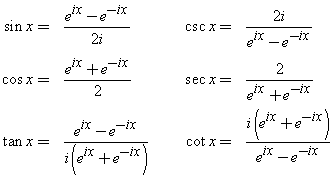# Dih3 与 S3

Standard

Dih3是3阶二面体群，S3是3阶置换群。Dih3 1 x x^2 S3 偶逆序 (1,2,3) (2,3,1) (3,2,1) Dih3 翻转 y x y x^2 y S3 奇逆序 (3,2,1) (1,3,2) (2,1,3)

# 指数函数族

Standardhttp://en.wikipedia.org/wiki/Inverse_trigonometric_functions

http://www.efunda.com/math/exp_log/exp2trig_hyper.cfm

Standard

# Dilworth 定理

Standard

Dilworth定理更加一般的阐述了这种对应的关系，接下来介绍这个定理：

Dilworth定理：集合中 最长的 反链长度  等于  把集合 链划分 的 最小 链个数。

# 拓扑空间（Topological Space）的定义

Standard# topo-

1.

a combining form meaning “place,” “local,” used in the formation of compound words:

topography; topology.
combining form of Greek tópos place, commonplace

1.任意S的元素（X的子集）的并依然属于S.
2.有限个S的元素的交依然属于S.
3.空集和X本身属于S

AB是R的一个子集，CD也是。那么（R, {AB,CD,CB,∅,R}）就是一个拓扑空间

A、B、C是R^2的一个子集（满足不等式(x-xi)^2+(y-yi)^2<=r^2）

http://dictionary.reference.com/browse/topo-?s=ts

# 任意多边形面积求法的证明

Standard

For any polygon, the area of it is half of the sum of the modulus of all adjacents points’ cross product in clockwise or counterclockwise.

proof:

Firstly, let we see how to compute the area of an enclosed curve (not cross itself) in 2-D.

For a ‘simple region’, we can compute its area using single variable directly. As for a complicated one, double integral can figure it out, or use green formula to convert to line integral.

formal proof:

PS: The rigorous defination of area see here.

Standard